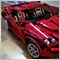# Need your help with iRSI67

My code work correctly with:
Momentum_Buffer [ Momentum_Position ] = Close [ Momentum_Position ] * 100 / Close [ Momentum_Position + Momentum_Period ] ;

But with:
Momentum_Buffer [ Momentum_Position ] = ( iRSI ( Symbol () , Period () , Momentum_Period , PRICE_MEDIAN , Momentum_Position ) * 100 ) / iRSI ( Symbol () , Period () , Momentum_Period , PRICE_MEDIAN , Momentum_Position + Momentum_Period ) ;

```//+------------------------------------------------------------------+
//|                                       Momentum Prime 12.12.4.mq4 |
//|                      Nguyen Xuan Nhat Huy & Nguyen Le Nguyen Huy |
//|                                                   29 - 03 - 2020 |
//+------------------------------------------------------------------+

//+--- Initation Set ---+

#property copyright "Nguyen Xuan Nhat Huy & Nguyen Le Nguyen Huy"

#property description "Momentum Prime 12.12.4"

#property indicator_separate_window
#property indicator_buffers 3

//+--- Input Indicator's Name ---+

extern string Indicator_Short_Name = "Momentum Prime 12.12.4" ;

//+--- Input Parameter ---+

extern int Momentum_Period = 7 ;

//+--- Input Parameter ---+

extern color Momentum_Color = clrRoyalBlue ;

//+--- Input Parameter ---+

extern int Level = 0 ;

//+--- Input Parameter ---+

extern color Level_Color = clrDimGray ;

//+--- Declare Variables ---+

int Momentum_Position ;
int Counted_Bars ;

//+--- Declare Variables ---+

double Momentum_Buffer [] ;
double Momentum_Buffer_High [] ;
double Momentum_Buffer_Low [] ;

//+--- Init Function ---+

void init ()
{
//+--- Set Indicator's Parameter ---+

IndicatorShortName ( Indicator_Short_Name ) ;

IndicatorDigits ( 4 ) ;
IndicatorBuffers ( 3 ) ;

//---- indicator line

SetIndexBuffer ( 0 , Momentum_Buffer ) ;
SetIndexLabel ( 0 , "Momentum Buffer" ) ;
//SetIndexStyle ( 0 , DRAW_HISTOGRAM , STYLE_SOLID , 2 , clrBlue ) ;
SetIndexStyle ( 0 , DRAW_NONE ) ;

SetIndexBuffer ( 1 , Momentum_Buffer_High ) ;
SetIndexLabel ( 1 , "Momentum High" ) ;
SetIndexStyle ( 1 , DRAW_HISTOGRAM , STYLE_SOLID , 2 , clrBlue ) ;

SetIndexBuffer ( 2 , Momentum_Buffer_Low ) ;
SetIndexLabel ( 2 , "Momentum Low" ) ;
SetIndexStyle ( 2 , DRAW_HISTOGRAM , STYLE_SOLID , 2 , clrRed ) ;

//SetIndexStyle ( 0 , DRAW_LINE , STYLE_SOLID , 2 , Momentum_Color ) ;

//+--- Set Level ---+

SetLevelValue ( 1 , Level ) ;

//+--- Set Level Style ---+

SetLevelStyle ( STYLE_DOT , 1 , Level_Color ) ;
}

//+--- Deinit Function ---+

void deinit ()
{
//+--- Delete Buffer ---+

SetIndexStyle ( 0 , DRAW_NONE ) ;
}

//+--- Start Function ---+

void start ()
{
//+--- Calculate Counted_Bars ---+

Counted_Bars = IndicatorCounted () ;

//+--- Bars <= Momentum_Period ---+

if ( Bars <= Momentum_Period )
{
deinit () ;
}

//+--- Set Buffer ---+

if ( Counted_Bars < 1 )
{
for ( Momentum_Position = 1 ; Momentum_Position <= Momentum_Period ; Momentum_Position ++ )
{
Momentum_Buffer [ Bars - Momentum_Position ] = EMPTY_VALUE ;
}
}

//+--- Calculate Momentum_Position ---+

Momentum_Position = Bars - Momentum_Period - 1 ;

//+--- Counted_Bars >= Momentum_Period ---+

if ( Counted_Bars >= Momentum_Period )
{
Momentum_Position = Bars - Counted_Bars - 1 ;
}

//+--- Momentum Function ---+

while ( Momentum_Position >= 0 )
{
Momentum_Buffer [ Momentum_Position ] = Close [ Momentum_Position ] * 100 / Close [ Momentum_Position + Momentum_Period ] ;

//Momentum_Buffer [ Momentum_Position ] = ( iRSI ( Symbol () , Period () , Momentum_Period , PRICE_MEDIAN , Momentum_Position ) * 100 ) / iRSI ( Symbol () , Period () , Momentum_Period , PRICE_MEDIAN , Momentum_Position + Momentum_Period ) ;

if ( Momentum_Buffer [ Momentum_Position ] >= 100 )
{
Momentum_Buffer_High [ Momentum_Position ] = Momentum_Buffer [ Momentum_Position ] - 100 ;
Momentum_Buffer_Low [ Momentum_Position ] = EMPTY_VALUE ;
}

if ( Momentum_Buffer [ Momentum_Position ] <= 100 )
{
Momentum_Buffer_High [ Momentum_Position ] = EMPTY_VALUE ;
Momentum_Buffer_Low [ Momentum_Position ] = Momentum_Buffer [ Momentum_Position ] - 100 ;
}

Momentum_Position -- ;
}
}

//+--- End ---+```1742

HuyTitan:

My code work correctly with:
Momentum_Buffer [ Momentum_Position ] = Close [ Momentum_Position ] * 100 / Close [ Momentum_Position + Momentum_Period ] ;

But with:
Momentum_Buffer [ Momentum_Position ] = ( iRSI ( Symbol () , Period () , Momentum_Period , PRICE_MEDIAN , Momentum_Position ) * 100 ) / iRSI ( Symbol () , Period () , Momentum_Period , PRICE_MEDIAN , Momentum_Position + Momentum_Period ) ;

This probably returned zero... so you should check for that, and skip the division if it's zero:

`iRSI ( Symbol () , Period () , Momentum_Period , PRICE_MEDIAN , Momentum_Position + Momentum_Period )`67

But logically, RSI of previous bar isn't zero.1742

HuyTitan:
But logically, RSI of previous bar isn't zero.

Your RSI period is 7, and you call iRSI() right up to Bars-1...20790

1. Why did you post your MT4 question in the Root / MT5 Indicators section instead of the MQL4 section, (bottom of the Root page?)
General rules and best pratices of the Forum. - General - MQL5 programming forum
Next time post in the correct place. The moderators will likely move this thread there soon.

2. The lookback of RSI is Momentum_Period - 1. You lookback Momentum_Position + Momentum_Period - 1 bars. Therefor your maximum lookback is the sum of those.
How to do your lookbacks correctly.

3. You should stop using the old event handlers and IndicatorCounted() and start using new event handlers.
Event Handling Functions - Functions - Language Basics - MQL4 Reference
How to do your lookbacks correctly.